Congruency - Different Criteria's

Congruence of Triangles
Serial order wise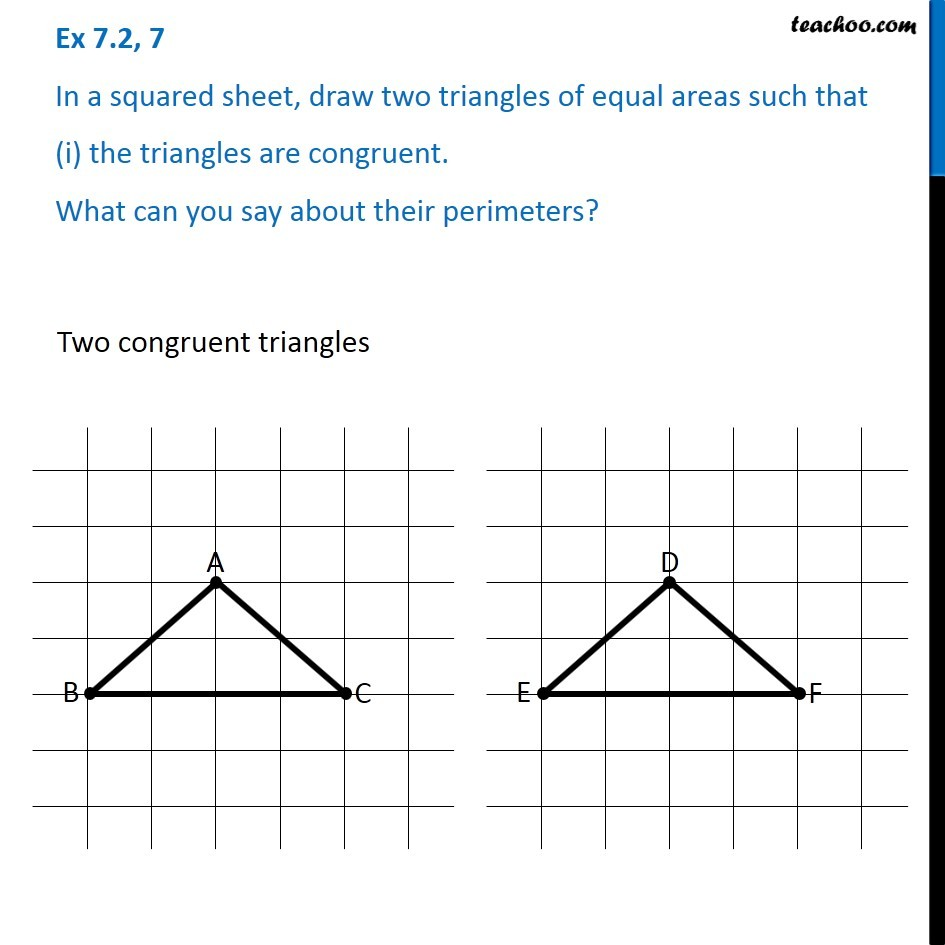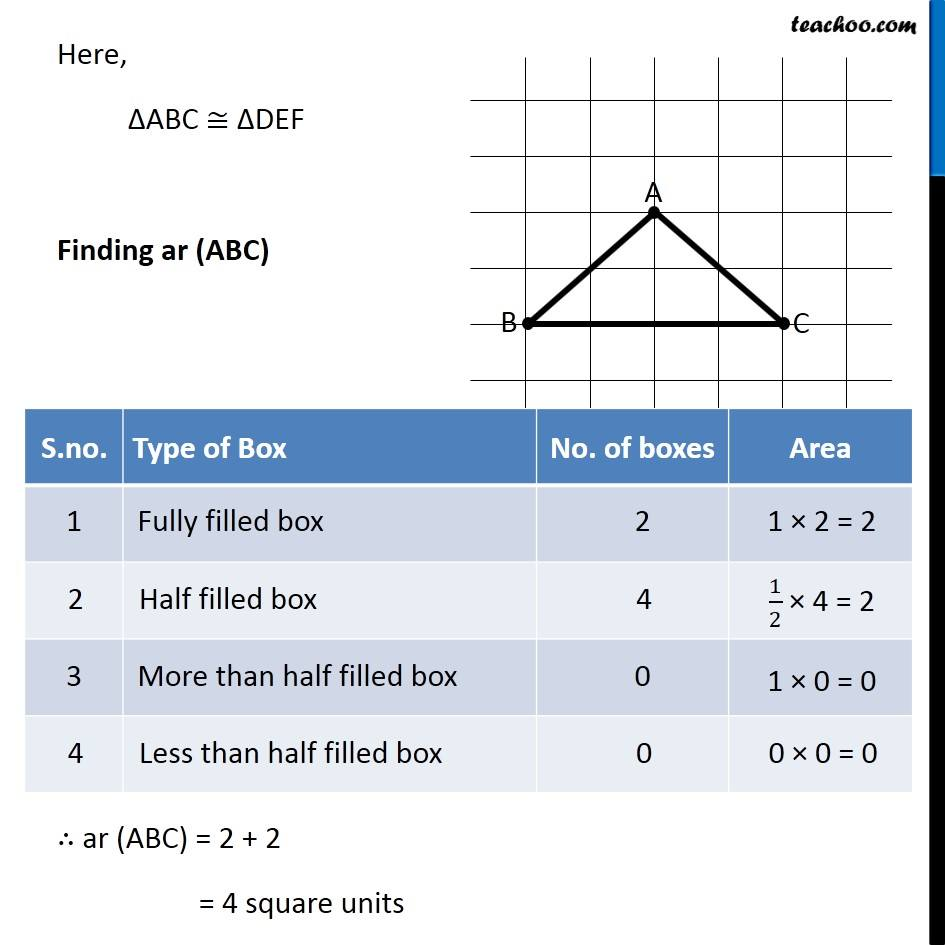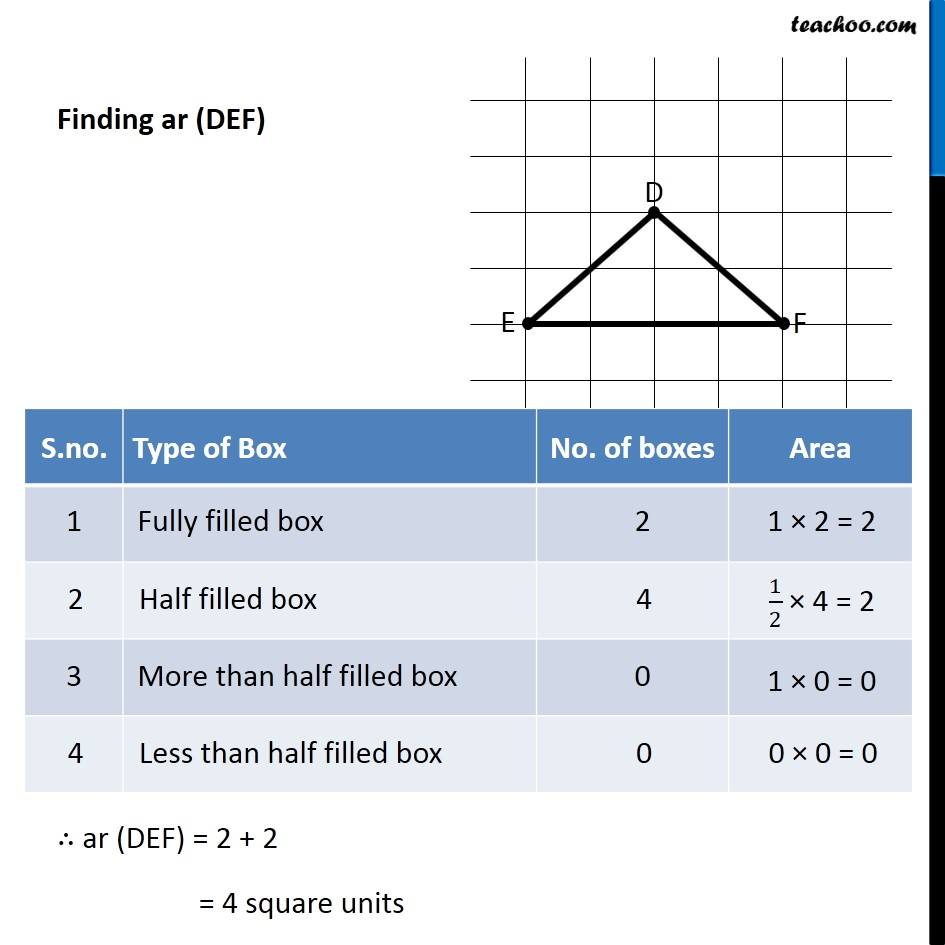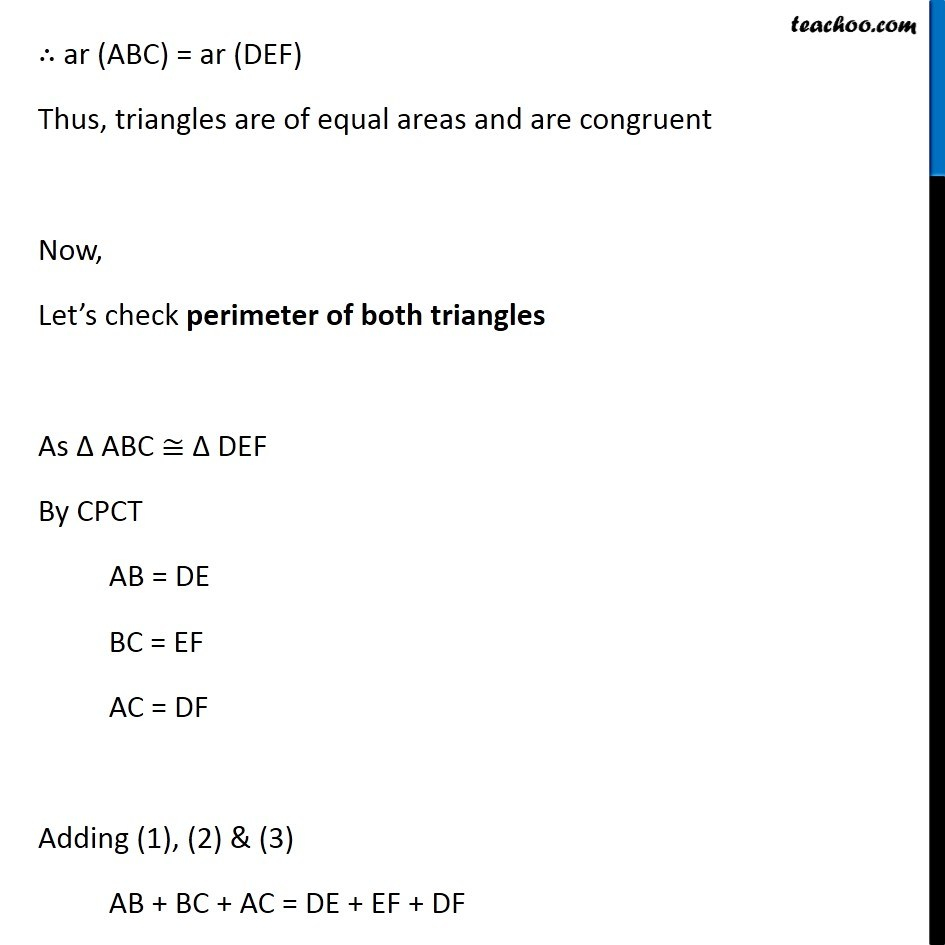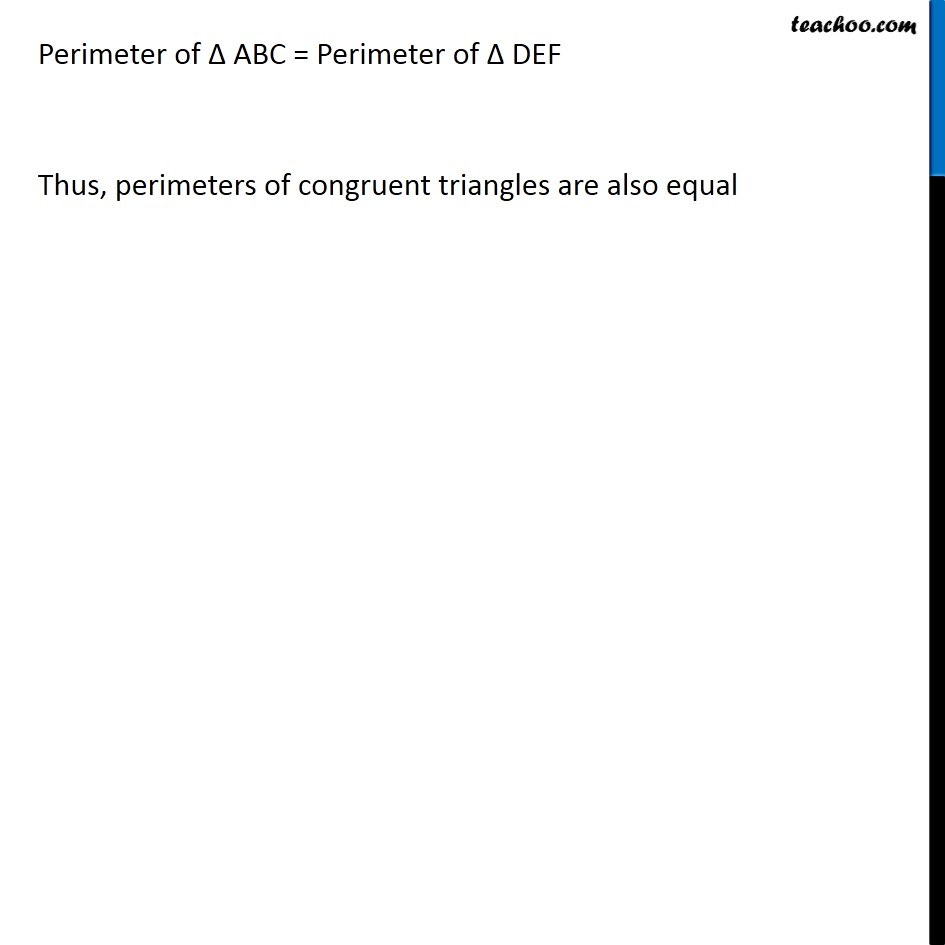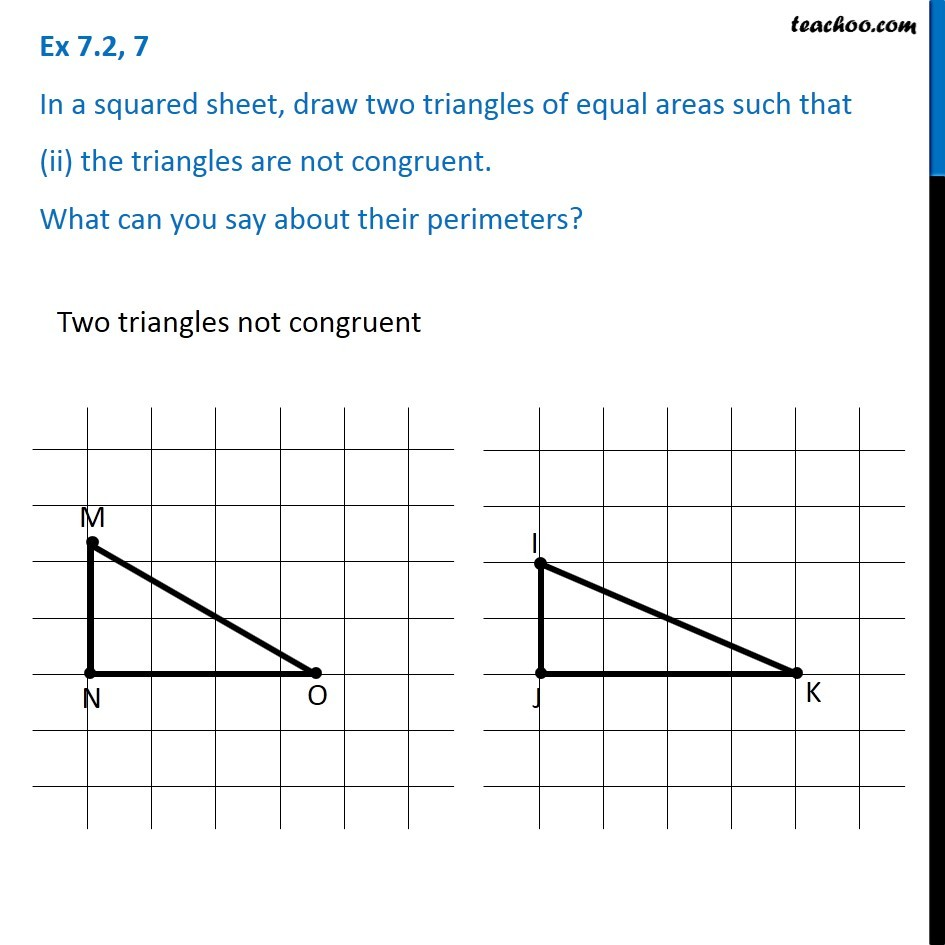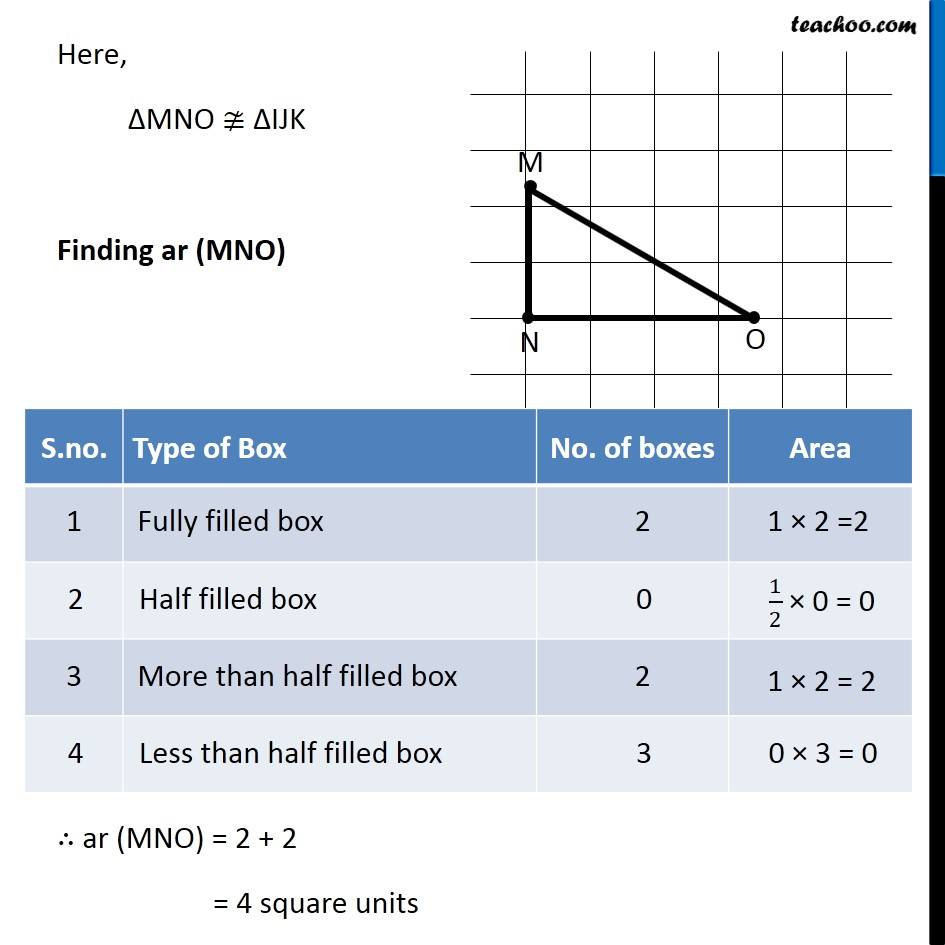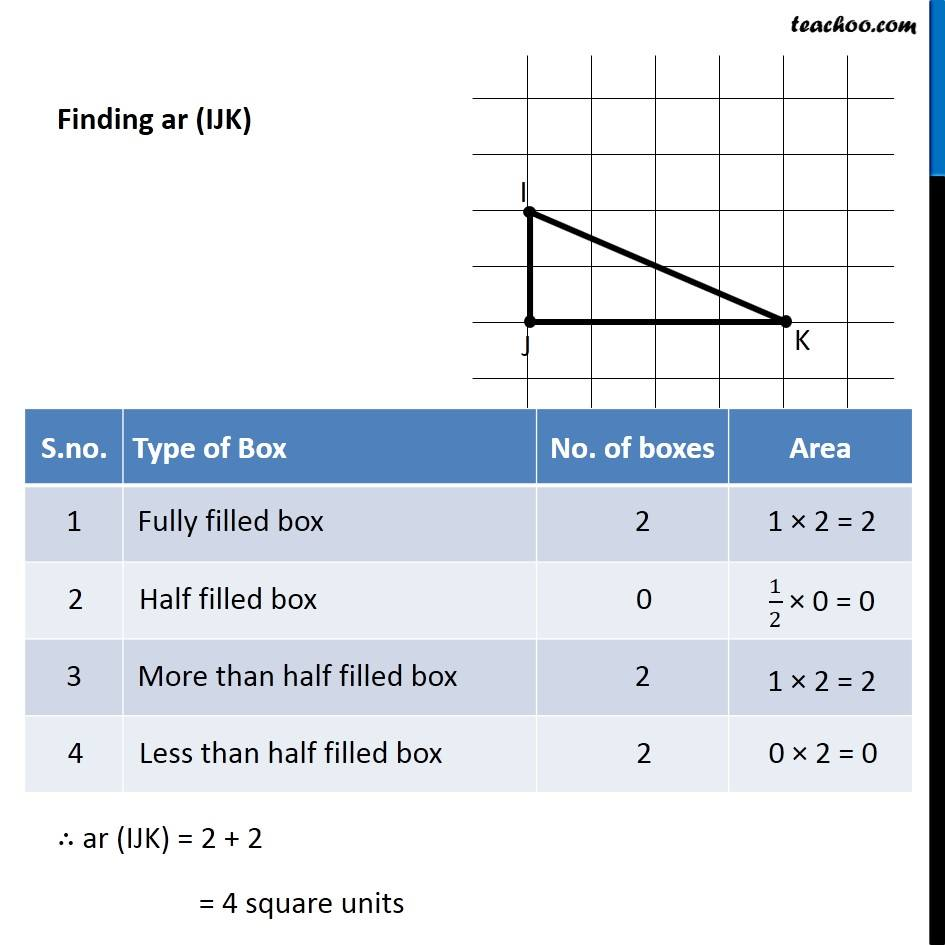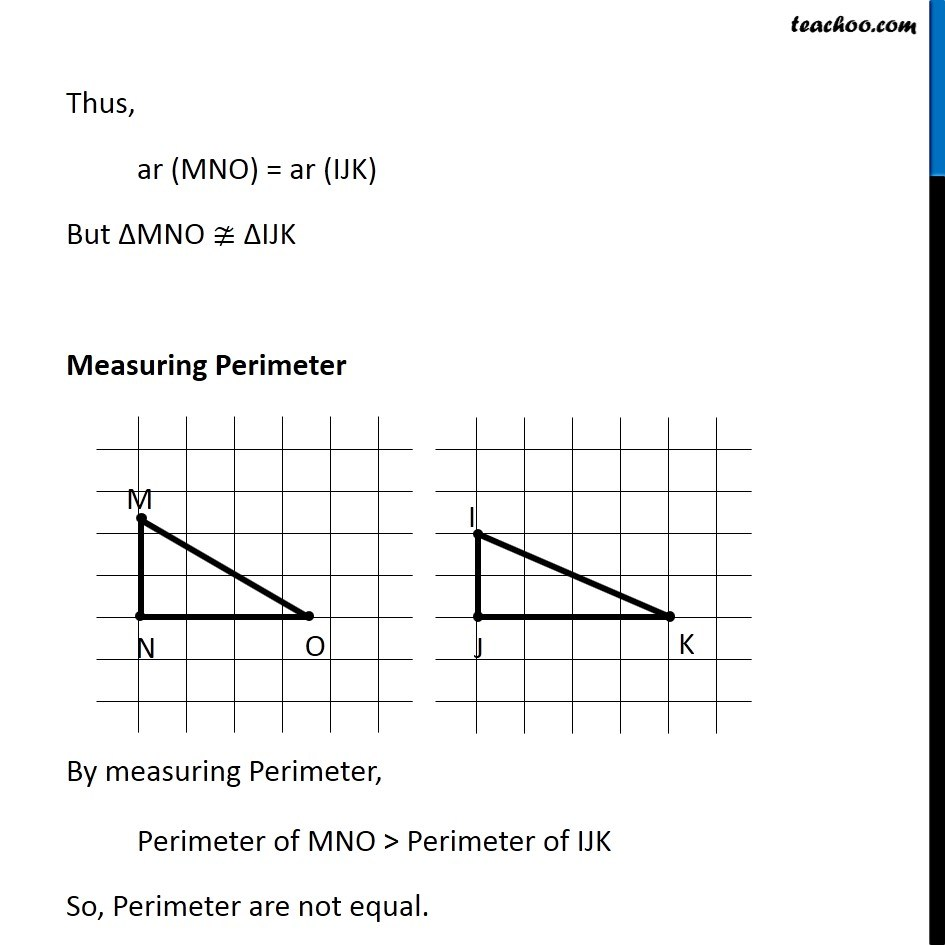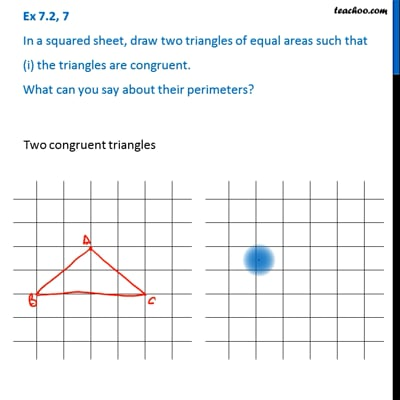This video is only available for Teachoo black users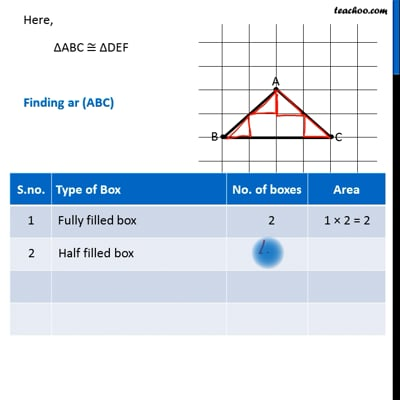This video is only available for Teachoo black users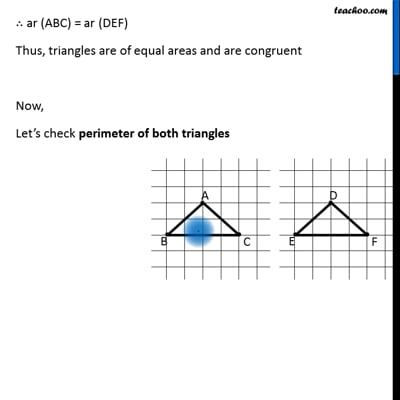This video is only available for Teachoo black users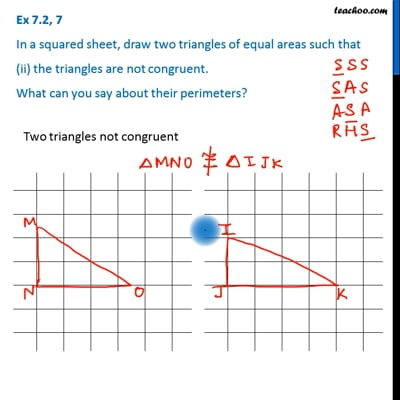This video is only available for Teachoo black users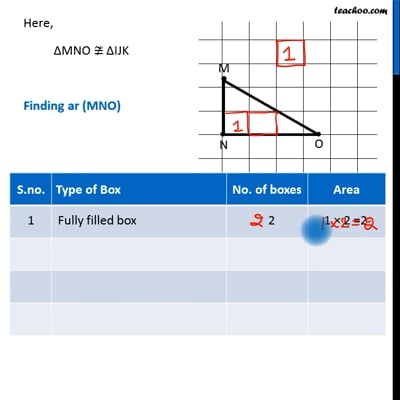This video is only available for Teachoo black users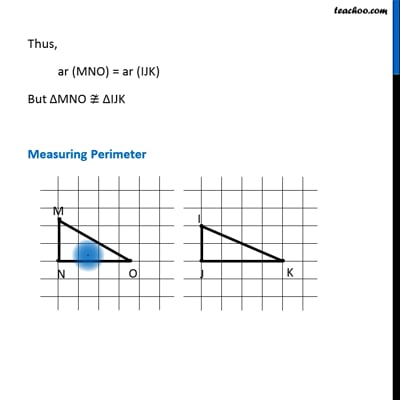This video is only available for Teachoo black users

Learn in your speed, with individual attention - Teachoo Maths 1-on-1 Class

### Transcript

Question 7 In a squared sheet, draw two triangles of equal areas such that (i) the triangles are congruent. What can you say about their perimeters?Two congruent triangles Here, ∆ABC ≅ ∆DEF Finding ar (ABC) ∴ ar (ABC) = 2 + 2 = 4 square units Finding ar (DEF) ∴ ar (DEF) = 2 + 2 = 4 square units ∴ ar (ABC) = ar (DEF) Thus, triangles are of equal areas and are congruent Now, Let’s check perimeter of both triangles As Δ ABC ≅ Δ DEF By CPCT AB = DE BC = EF AC = DF Adding (1), (2) & (3) AB + BC + AC = DE + EF + DF Perimeter of Δ ABC = Perimeter of Δ DEF Thus, perimeters of congruent triangles are also equal Question 7 In a squared sheet, draw two triangles of equal areas such that (ii) the triangles are not congruent. What can you say about their perimeters? Two triangles not congruent Here, ∆MNO ≇ ∆IJK Finding ar (MNO) ∴ ar (MNO) = 2 + 2 = 4 square units Finding ar (IJK) ∴ ar (IJK) = 2 + 2 = 4 square units Thus, ar (MNO) = ar (IJK) But ∆MNO ≇ ∆IJK Measuring Perimeter By measuring Perimeter, Perimeter of MNO > Perimeter of IJK So, Perimeter are not equal.